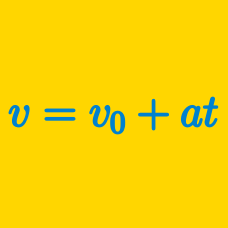Classical Mechanics

# Position Vector for Constant Acceleration

If an object is thrown straight upward with an initial speed of $8\text{ m/s}$ and takes $7$ seconds to hit the ground, from what height was the object thrown?

Assume that gravitational acceleration is $g=10\text{ m/s}^2.$

A race car is on a straight track. The race car accelerates from rest, covering $100~\mbox{m}$ in $5~\mbox{seconds}$. What is the acceleration of the race car in $\mbox{m/s}^2$?

Details and assumptions

• You may neglect air resistance.

A rock is dropped vertically off the edge of a cliff and hits the ground with an impact velocity of $90\text{ m/s}.$ How high is the cliff?

The gravitational acceleration is $g=10\text{ m/s}^2.$

An object initially at rest starts moving in a straight line, accelerating uniformly at $3\text{ m/s}^2$ for $2$ seconds. Then it moves with a constant acceleration of $-2\text{ m/s}^2$ for $8$ seconds, and then it accelerates uniformly at $1\text{ m/s}^2$ for $8$ seconds. Find the displacement of the object during the total $18$ seconds.

Cliff diving is an extreme sport. If a cliff diver dives from a platform 50 meters above the water, how long does the dive take in seconds?

Details and assumptions

• The acceleration of gravity is $-9.8~\mbox{m/s}^2$.
• The diver can be treated as a point mass and has no vertical velocity at the start of the dive.
• Ignore air resistance.
×